Map

Map and (f[#]&) can be used to map any function (f) to each argument under  any Head.  In the next Cell (Exp[_]) mapped to each term in a sum.  Many  users understand what Map does with the default level specification.  In the  first example the default level specification is used, and Exp[_] is mapped  to each expression at the first level.  The #& notation is explained in the  discussion of Function.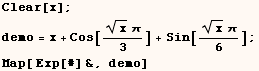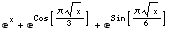Note:  The shorthand notation for Map is   /@ .  This shorthand notation is
used to do the same as the previous line.  At first this notation seems very
cryptic.If you need to specify a level specification the short hand notation is  not at all convenient.  Different variations of level specification are demonstrated in the lines below.  Many other commands allow a user to  specify the level specification, and the conventions are always the same.   Level specification {3} means to only map the function to Level 3.  In the  next Cell we Map
(# + 1)& to all subexpressions in (demo) at Level {3}.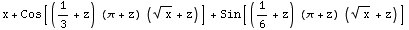Level specification {-1} refers to the smallest subexpressions (the  atoms).  In the next cell we add (1) to each atom in (demo).  It should be  pointed out that the list of atoms includes 1/6, 1/3,  and 1/2, instead of 1,  2, 3, 6.  This is because since Mathematica rational numbers as atoms.  Complex numbers are also considered atoms.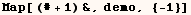Level specification (2) refers to all levels from (Level 1) to (Level 2).
Now we Map ( #/z&) to all subexpressions of (demo) from (Level 1) to (Level
2).The next line Maps (#+z&) to all subexpressions of (demo) at (Level 2) and
(Level 3).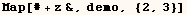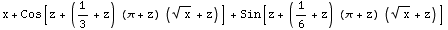Heads Option

Map has a Heads option like several other functions, but it's hard to think
of a practical use for this feature.  By default Map uses the setting (Heads
→False).  If your writing a program and you need to make it full proof
you should use the form  Map[f, expr, Heads→False]  instead of the
shorter (and normally equivalent) form  f/@expr.  The reason is that the user
may have changed the default setting via SetOptions[Map, Heads→True]
which would change the behavior of  f/@expr.  Now consider the next cell to
see how Map uses the Heads option.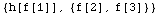In the cell above (f) was mapped to expressions at positions {1,1}, {2,1},
and {2,2} since they are all the expressions at level 2.  In the next cell
the same example is evaluated with the setting (Heads→True) and (f) is
also mapped to every head at level 2. In this case the heads at level 2 are
(h) at position {1,0} and List at position {2,0}.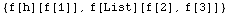Created by Mathematica  (May 16, 2004)

Back to Ted’s Tricks index page